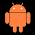## Wednesday, December 2, 2015

### Random projection in a nutshell

I was reading the random projection paper – written by Teija Seitola et al. – the other day, and I think I have now quite a good understanding of how the method works.

The whole thing is based on Johnson–Lindenstrauss lemma from 1984. The lemma says that random projection (nearly) preserves the distances.

Let X be a n×d-dimensional data matrix, where n is the number of data (or ensemble) points and d is the actual dimension. Let f be d×k-dimensional random projection matrix. Let xi and xj be d-dimensional data vectors and ε>0. Now we have that

(1-ε)×ǁxi-xjǁ2 ≤ ǁf(xi)-f(xj2 ≤ (1+ε)×ǁxi-xjǁ2.

In the paper there are also to explicit formulas for k that surprisingly do not depend on the dimension d, but only on the number of observations n and the error ε. For example, k > 4×(ε2/2-ε3/3)-1×log(n).

So if, for example in correlation dimension, we set n = 2000 and ε = 0.1, we need k that is higher than 4*(.1^2/2-.1^3/3)^(-1)*log(2000) = 6515.1. I tested this with 320-dimensional Lorenz and set k to 10000 and the thing works since the curves became so similar (see figure below).

As a conclusion, I would say that random projection method works “better” in large than small dimensional problems, and that the dimension have to be really large before it is worth to use random projections in applications where the preservation of distances is very very important. Note that there are other kinds of applications too…

Below, there is a little Matlab example.

Ciao,
Janne

%% Random projection Matlab example

n = 2000; % Number of time points
d = 20000; % Dimension
k = 10000; % Random dimension

X = 10+5*randn(n,d); % Data matrix

R = randn(d,k); % Random projection matrix
for kkk = 1:d
R(kkk,:) = R(kkk,:)/norm(R(kkk,:)); % Normalization
end

P = X*R; % Projection

% Distances
ii = 10; jj = 20;
norm(X(ii,:)-X(jj,:))

norm(P(ii,:)-P(jj,:))

1.2.